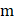# A uniform sphere of massradiusand moment of inertiaabout its centre moves along the-axis as shown in Figure. Its centre of mass moves with velocityand it rotates about its centre of mass with angular velocity.Let. The angular momentum of the body about the originisa)only ifb) Greater thanifc) Less thanifd)for all values ofand## Question ID - 100208 :- A uniform sphere of massradiusand moment of inertiaabout its centre moves along the-axis as shown in Figure. Its centre of mass moves with velocityand it rotates about its centre of mass with angular velocity.Let. The angular momentum of the body about the originisa)only ifb) Greater thanifc) Less thanifd)for all values ofand3537

(d)which is constant)

Next Question :
 A force exerts an impulseon a particle changing its speed from initial velocityto final velocity 2The applied force and the initial velocity are oppositely oriented along the same line. The work done by the force is a)b)c)d)